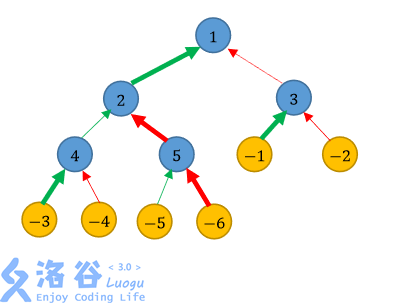P4438 [HNOI/AHOI2018]道路

• 799通过
• 2.1K提交
• 题目提供者 Kelin
• 评测方式 云端评测
• 标签 动态规划,动规,dp 枚举,暴力 树形动规 概率论,统计 深度优先搜索,DFS 各省省选 2018 安徽 湖南
• 难度 提高+/省选-
• 时空限制 1000ms / 512MB
• 提示：收藏到任务计划后，可在首页查看。

题目描述

W 国的交通呈一棵树的形状。W 国一共有$n - 1$个城市和$n$个乡村，其中城市从$1$到$n - 1$ 编号，乡村从$1$到$n$编号，且$1$号城市是首都。道路都是单向的，本题中我们只考虑从乡村通往首都的道路网络。对于每一个城市，恰有一条公路和一条铁路通向这座城市。对于城市i， 通向该城市的道路（公路或铁路）的起点，要么是一个乡村，要么是一个编号比$i$大的城市。 没有道路通向任何乡村。除了首都以外，从任何城市或乡村出发只有一条道路；首都没有往 外的道路。从任何乡村出发，沿着唯一往外的道路走，总可以到达首都。

W 国的国王小 W 获得了一笔资金，他决定用这笔资金来改善交通。由于资金有限，小 W 只能翻修$n - 1$条道路。小 W 决定对每个城市翻修恰好一条通向它的道路，即从公路和铁 路中选择一条并进行翻修。小 W 希望从乡村通向城市可以尽可能地便利，于是根据人口调 查的数据，小 W 对每个乡村制定了三个参数，编号为$i$的乡村的三个参数是$a_i$，$b_i$和$c_i$。假设 从编号为$i$的乡村走到首都一共需要经过$x$条未翻修的公路与$y$条未翻修的铁路，那么该乡村 的不便利值为

$$c_i \cdot (a_i + x) \cdot (b_i + y)$$

在给定的翻修方案下，每个乡村的不便利值相加的和为该翻修方案的不便利值。 翻修$n - 1$条道路有很多方案，其中不便利值最小的方案称为最优翻修方案，小 W 自然 希望找到最优翻修方案，请你帮助他求出这个最优翻修方案的不便利值。

输入输出格式

输入格式：

第一行为正整数$n$。

接下来$n - 1$行，每行描述一个城市。其中第$i$行包含两个数$s_i,t_i$。$s_i$表示通向第$i$座城市 的公路的起点，$t_i$表示通向第i座城市的铁路的起点。如果$s_i > 0$，那么存在一条从第$s_i$座城 市通往第$i$座城市的公路，否则存在一条从第$-s_i$个乡村通往第i座城市的公路；$t_i$类似地，如 果$t_i > 0$，那么存在一条从第$t_i$座城市通往第i座城市的铁路，否则存在一条从第$-t_i$个乡村通 往第$i$座城市的铁路。

接下来$n$行，每行描述一个乡村。其中第i行包含三个数$a_i,b_i,c_i$，其意义如题面所示。

输出格式：

输出一行一个整数，表示最优翻修方案的不便利值。

输入输出样例

输入样例#1： 复制
6
2 3
4 5
-1 -2
-3 -4
-5 -6
1 2 3
1 3 2
2 1 3
2 3 1
3 1 2
3 2 1
输出样例#1： 复制
54
输入样例#2： 复制
9
2 -2
3 -3
4 -4
5 -5
6 -6
7 -7
8 -8
-1 -9
1 60 1
1 60 1
1 60 1
1 60 1
1 60 1
1 60 1
1 60 1
1 60 1
1 60 1
输出样例#2： 复制
548
输入样例#3： 复制
12
2 4
5 3
-7 10
11 9
-1 6
8 7
-6 -10
-9 -4
-12 -5
-2 -3
-8 -11
53 26 491
24 58 190
17 37 356
15 51 997
30 19 398
3 45 27
52 55 838
16 18 931
58 24 212
43 25 198
54 15 172
34 5 524
输出样例#3： 复制
5744902



说明

【样例解释 1】如图所示，我们分别用蓝色、黄色节点表示城市、乡村；用绿色、红色箭头分别表示 公路、铁路；用加粗箭头表示翻修的道路。

一种不便利值等于54的方法是：翻修通往城市2和城市5的铁路，以及通往其他城市的 公路。用→和⇒表示公路和铁路，用∗→和∗⇒表示翻修的公路和铁路，那么：

编号为1的乡村到达首都的路线为：-1 ∗→ 3 ⇒ 1，经过0条未翻修公路和1条未翻修铁 路，代价为3 × (1 + 0) × (2 + 1) = 9；
编号为2的乡村到达首都的路线为：-2 ⇒ 3 ⇒ 1，经过0条未翻修公路和2条未翻修铁 路，代价为2 × (1 + 0) × (3 + 2) = 10；
编号为3的乡村到达首都的路线为：-3 ∗→ 4 → 2 ∗→ 1，经过1条未翻修公路和0条未 翻修铁路，代价为3 × (2 + 1) × (1 + 0) = 9；
编号为4的乡村到达首都的路线为：-4 ⇒ 4 → 2 ∗→ 1，经过1条未翻修公路和1条未翻 修铁路，代价为1 × (2 + 1) × (3 + 1) = 12；
编号为5的乡村到达首都的路线为：-5 → 5 ∗⇒ 2 ∗→ 1，经过1条未翻修公路和0条未 翻修铁路，代价为2 × (3 + 1) × (1 + 0) = 8；
编号为6的乡村到达首都的路线为：-6 ∗⇒ 5 ∗⇒ 2 ∗→ 1，经过0条未翻修公路和0条未翻修铁路，代价为1 × (3 + 0) × (2 + 0) = 6；

总的不便利值为9 + 10 + 9 + 12 + 8 + 6 = 54。可以证明这是本数据的最优解。

【样例解释 2】

在这个样例中，显然应该翻修所有公路。

【数据范围】 一共20组数据，编号为1 ∼ 20。 对于编号$\le 4$的数据，$n \le 20$；
对于编号为5 ∼ 8的数据，$a_i,b_i,c_i \le 5$，$n \le 50$；
对于编号为9 ∼ 12的数据，$n \le 2000$；
对于所有的数据，$n \le 20000$，$1 \le a_i,b_i \le 60$，$1 \le c_i \le 10^9$，$s_i,t_i$是$[-n,-1] \cup (i,n - 1]$内的整数，任意乡村可以通过不超过40条道路到达首都。

提示
标程仅供做题后或实在无思路时参考。
请自觉、自律地使用该功能并请对自己的学习负责。
如果发现恶意抄袭标程，将按照I类违反进行处理。